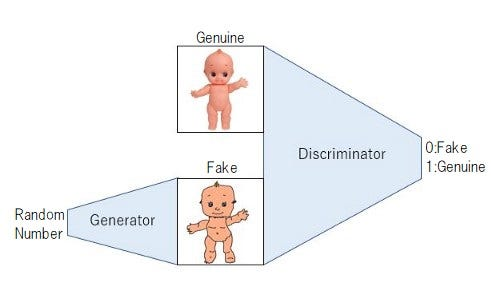# 3 minutes for cooking DCGAN

Source: Deep Learning on Medium# 3 minutes for cooking DCGAN

Today, I’m going to cook a GAN(Image Generation Algorithm) that everyone likes. The target image is generated from an appropriate random number. So it’s perfect for busy days! Bitterness(noise) is also reduced by adding a convolution layer to the secret ingredient. How about the standard for the coming season?

# Material (batch_size portion)

① noise: 10 (for 28×28 images). Create with random numbers.
② Genuine Image: (height, width, channel) format.
③ Generator model: 1 (input->noise, output ->(height, width, channel)). Used to generate an image from noise.
④ Discriminator model: 1(input ->(height, width, channel), output ->0 or 1). Used to determine whether the image is fake or genuine.

# Pretreatment

## Generator model

The last is tanh and -1<outputs<1, enlarging to 28×28 while performing deconvolution.

`inputs = Input((10,))fc1 = Dense(input_dim=10, units=128*7*7)(inputs)fc1 = BatchNormalization()(fc1)fc1 = LeakyReLU(0.2)(fc1)fc2 = Reshape((7, 7, 128), input_shape=(128*7*7,))(fc1)up1 = Conv2DTranspose(64, (2, 2), strides=(2, 2), padding=’same’)(fc2)conv1 = Conv2D(64, (3, 3), padding=’same’)(up1)conv1 = BatchNormalization()(conv1)conv1 = Activation(‘relu’)(conv1)up2 = Conv2DTranspose(64, (2, 2), strides=(2, 2), padding=’same’)(conv1)conv2 = Conv2D(1, (5, 5), padding=’same’)(up2)outputs = Activation(‘tanh’)(conv2)model = Model(inputs=[inputs], outputs=[outputs]`

## Discriminator model

It’s a basic two-class classification model . I think that even cooking beginners can make it with peace of mind.

`inputs = Input((28, 28, 1))conv1 = Conv2D(64, (5, 5), padding=’same’)(inputs)conv1 = LeakyReLU(0.2)(conv1)pool1 = MaxPooling2D(pool_size=(2, 2))(conv1)conv2 = Conv2D(128, (5, 5), padding=’same’)(pool1)conv2 = LeakyReLU(0.2)(conv2)pool2 = MaxPooling2D(pool_size=(2, 2))(conv2)fc1 = Flatten()(pool2)fc1 = Dense(1)(fc1)outputs = Activation(‘sigmoid’)(fc1)model = Model(inputs=[inputs], outputs=[outputs])`

# Cooking(train) procedure

1. In advance, the generator model and discriminator model are compiled. The output label of discriminator is 0=fake, and 1=genuine.
2. Connect the two models with generator -> discriminator to create a `d_on_g` model (input -> noise, output -> 0 or 1).
3. The noise is made from uniform random number between 0 and 1.
4. Add the noise created in 3 to the generator model and create a generator_image(batch_size, height, width, channel). When the generator model is optimized, the fake image that looks like a genuine one will be output here.
5. Mix the genuine image and the generator_image created in 4, and create X=(batch_size×2, height, width, channel) and y = (1,1,…,1,0,0,…,0), these are images(X) and the correct answer labels of X.
6. Set trainable = True for discriminator model.
7. Set X and Y of 5 as a a set, and update the gradient of the discriminator model once. If you use keras, the code is `train_on_batch(X,y)` .
8. After that, set the trainable of discriminator model to False, add noise to d_on_g model created in 2, and update gradient once (that is, only generator model is updated). If you use keras, the code is `train_on_batch(noise, label)` . At this time, all the labels of generator_images are set to 1.***
9. Repeat 3~8 many times. If the loss of 7 is small enough (discriminator model can distinguish fake and genuine) and 8 is large ( the “1” label attached to the generated image is recognized to be different), it is complete!
• ** Reason for setting the generated image label to “1” in 8: The purpose of the generator model is to generate a real image. Therefore, the generator evolves to generate genuine-like images when learning the identification result as genuine.